# 从赌钱游戏看 PageRank 算法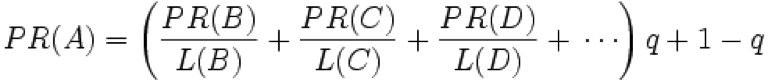double x1=1.0,x2=1.0,x3=1.0;

double x2 income =x1/2.0;

double x3
income =x1/2.0+x2;

double x1_ income =x3;

// Gamble 单机程序
public class Gamble
{
public static double x1=1.0,x2=1.0,x3=1.0;

public static void playgame(){
double x2_income=x1/2.0;        double x3_income=x1/2.0+x2;        double x1_income=x3;        x1=x1_income;        x2=x2_income;        x3=x3_income;        System.out.println("x1:"+x1+", x2:"+x2+", x3:"+x3); }                public static void main(String[] args){        for(int i=0;i<500;i++){        System.out.print("第"+i+"轮 ");        playgame();        } } }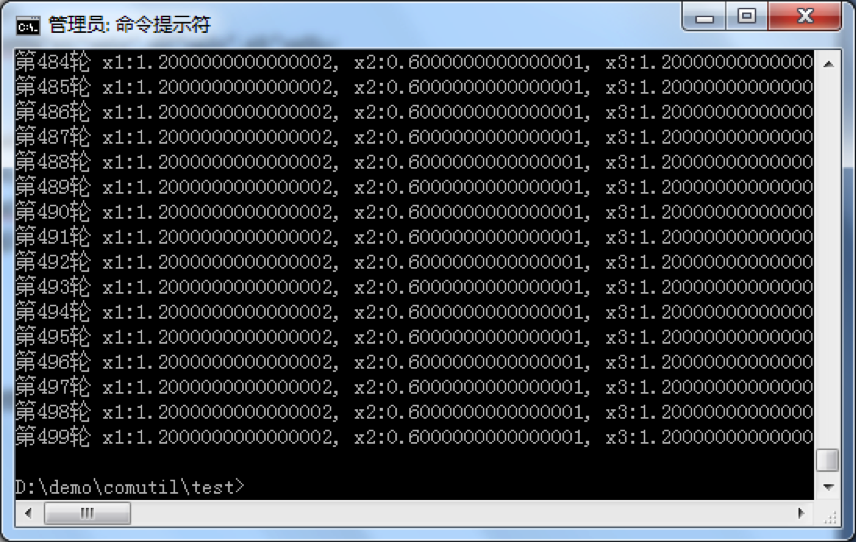x1:1.2000000000000002, x2:0.6000000000000001, x3:1.2000000000000002

…...

double x2_income=x1/2.0+x3/2.0;

double x3_income=x1/2.0+x2;

double x1_income=x3/2.0;

x1:0.6666666666666667, x2:1.0, x3:1.3333333333333333

double x2_income=x1/2.0+x3/2.0;

double x3_income=x1/2.0+x2;

double x1_income=0;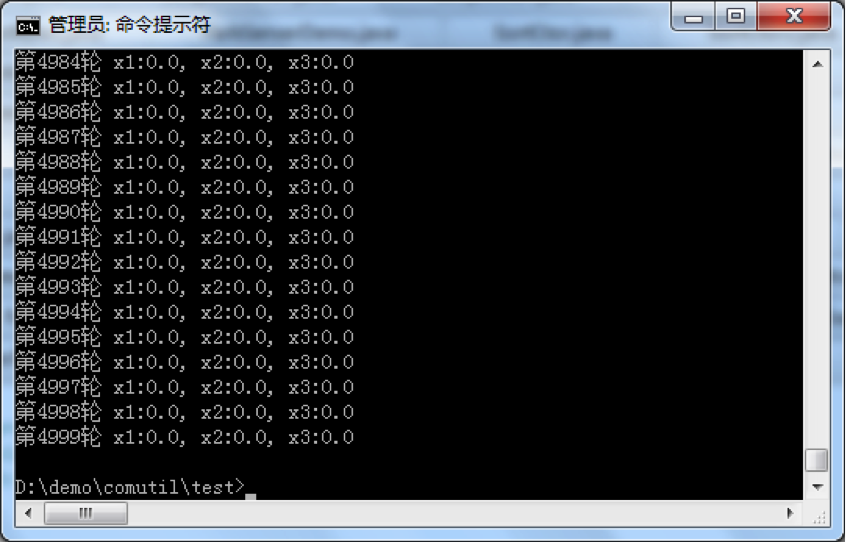A 包含 B 和 C 的链接

B 包含 C 的链接

C 包含 A 的链接

PR(B)=PR(A)/2;

PR(B)=PR(A)/2+PR(C);

PR(A)=PR(C);

PR(x1)+ PR(x2)+ …+PR(xn)

=( (PR(x2)/ L(x2)+ … )q+1-q) + … + ( (PR(x1)/ L(x1)+ … )q+1-q)

=(PR(x1) L(x1)/L(x1) + PR(x2) L(x2)/L(x2) + … + PR(xn) L(xn)/L(xn))q + n(1-q)

=( PR(x1) + PR(x2) + … + PR(xn))
q + n - nq

=n
q + n – n*q

= n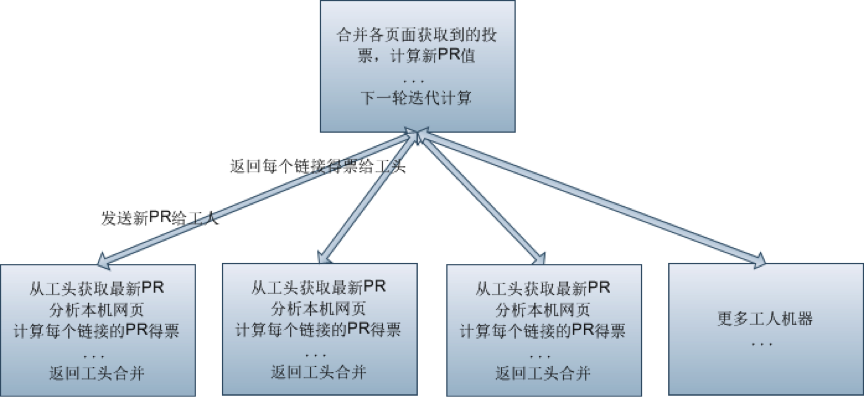#### 程序实现：

PageRankWorker：是一个 PageRank 工人实现，为了方便演示，它通过一个字符串数组代表包括的链接（实际上应该从本地网页文件里获取）

pagepr = pagepr+(Double)prwh.getObj(page);

#### 运行步骤：

1、  启动 ParkServerDemo（它的 IP 端口已经在配置文件指定）

java -cp fourinone.jar; ParkServerDemo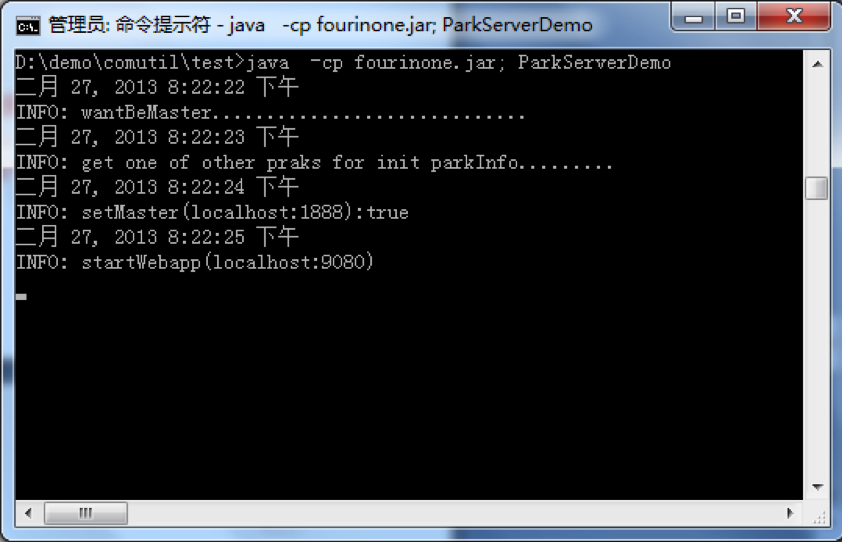2、运行 A、B、C 三个 PageRankWorker，传入不同的 IP 和端口号

java  -cp fourinone.jar; PageRankWorker localhost 2008 A

java  -cp fourinone.jar; PageRankWorker localhost 2009 B

java  -cp fourinone.jar; PageRankWorker localhost 2010 C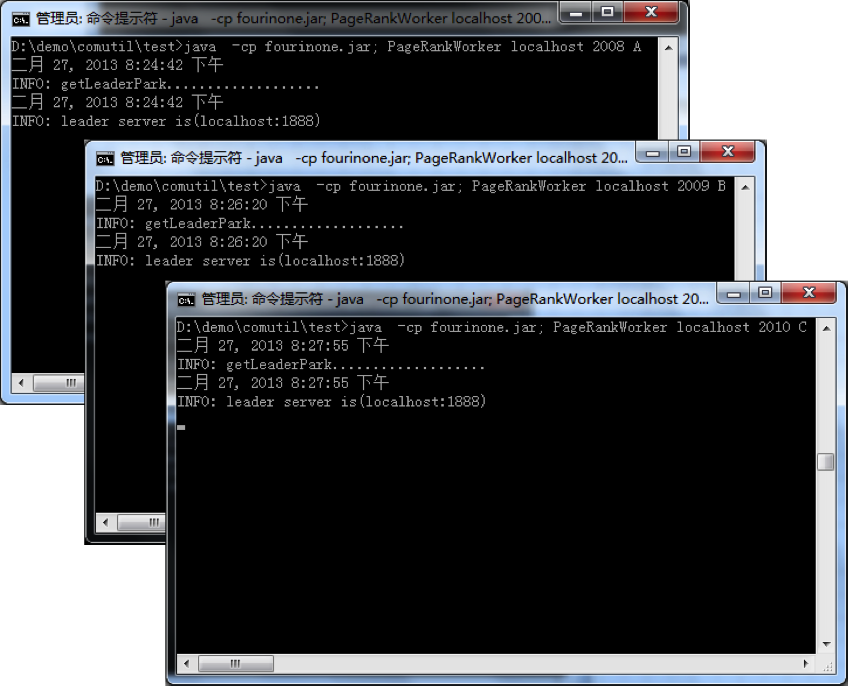3、运行 PageRankCtor

java -cp fourinone.jar; PageRankCtor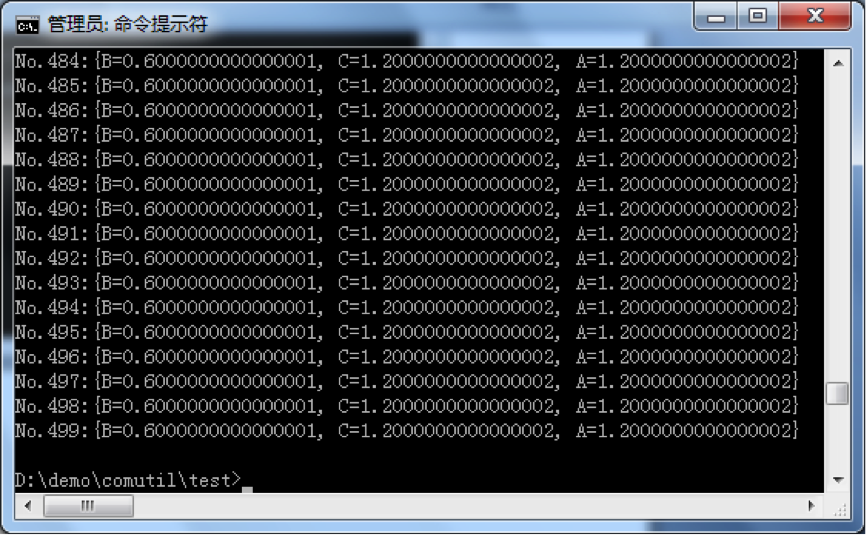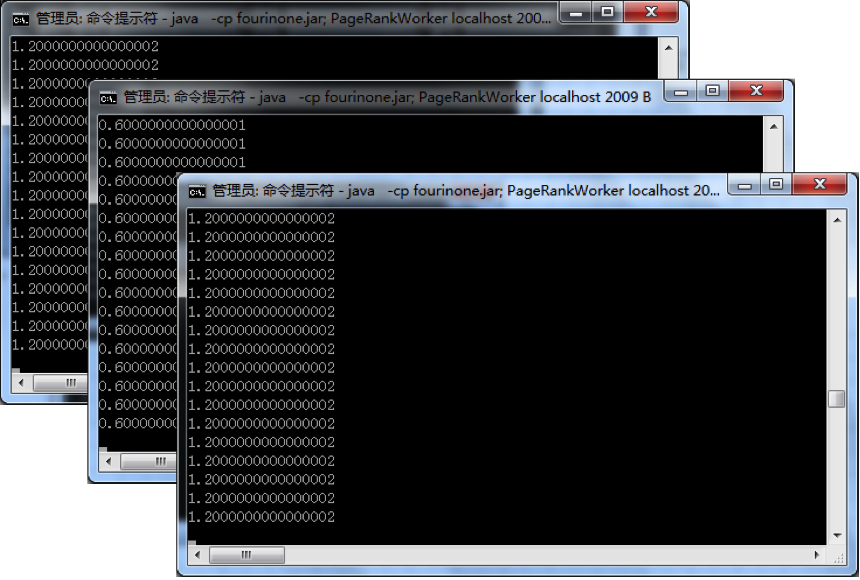// ParkServerDemo
import com.fourinone.BeanContext;
public class ParkServerDemo{
public static void main(String[] args){
BeanContext.startPark();
}
}// PageRankWorker import com.fourinone.MigrantWorker; import com.fourinone.WareHouse; import com.fourinone.Workman;public class PageRankWorker extends MigrantWorker{        public String page = null;        public String[] links = null;        public PageRankWorker(String page, String[] links){               this.page = page;               this.links = links;        }              public WareHouse doTask(WareHouse inhouse) {               Double pr = (Double)inhouse.getObj(page);               System.out.println(pr);               WareHouse outhouse = new WareHouse();               for(String p:links)                   outhouse.setObj(p, pr/links.length);// 对包括的链接 PR 投票                  return outhouse;        }        public static void main(String[] args){               String[] links = null;               if(args.equals("A"))                   links = new String[]{"B","C"};//A 页面包括的链接               else if(args.equals("B"))                   links = new String[]{"C"};               else if(args.equals("C"))                   links = new String[]{"A"};                                               PageRankWorker mw = new PageRankWorker(args,links);               mw.waitWorking(args,Integer.parseInt(args),"pagerankworker");         } }// PageRankCtor import com.fourinone.Contractor; import com.fourinone.WareHouse; import com.fourinone.WorkerLocal; import java.util.Iterator;public class PageRankCtor extends Contractor{        public WareHouse giveTask(WareHouse inhouse){               WorkerLocal[] wks = getWaitingWorkers("pagerankworker");               System.out.println("wks.length:"+wks.length);                                    for(int i=0;i<500;i++){//500 轮                   WareHouse[] hmarr = doTaskBatch(wks, inhouse);                   WareHouse prwh = new WareHouse();                       for(WareHouse result:hmarr){                           for(Iterator iter=result.keySet().iterator();iter.hasNext();){                               String page = (String)iter.next();                               Double pagepr = (Double)result.getObj(page);                               if(prwh.containsKey(page))                                   pagepr = pagepr+(Double)prwh.getObj(page);                                   prwh.setObj(page,pagepr);                             }                         }                    inhouse = prwh;// 迭代                    System.out.println("No."+i+":"+inhouse);                 }                 return inhouse;               }                public static void main(String[] args){              PageRankCtor a = new PageRankCtor();              WareHouse inhouse = new WareHouse();              inhouse.setObj("A",1.00d);//A 的 pr 初始值              inhouse.setObj("B",1.00d);//B 的 pr 初始值              inhouse.setObj("C",1.00d);//C 的 pr 初始值              a.giveTask(inhouse);              a.exit();        } }